Algebra 2 2-6 Guided Practice: Families of Functions
starstarstarstarstarstarstarstarstarstar
by Matthew Richardson
| 23 Questions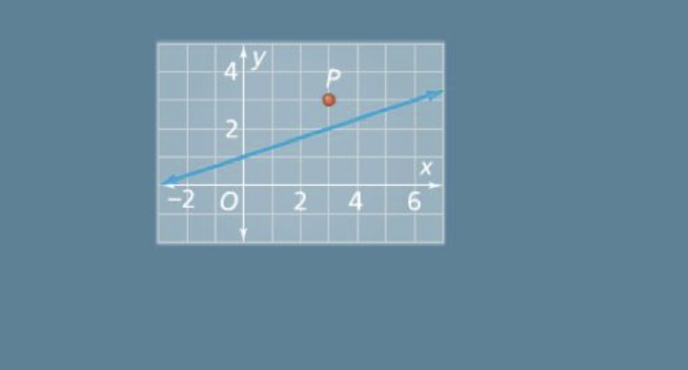a
1
2
Solve It! The equation of the line is:

1
10
How could you change the y-intercept so the graph of a second equation passes through point P?
Increase the y-intercept by 1 to form the equation:
Decrease the y-intercept by 1 to form the equation:
2
1
How could you change the slope so the graph of a second equation passes through point P?
Increase the slope to 2/3 to form the equation:
Decrease the slope to 1/6 to form the equation: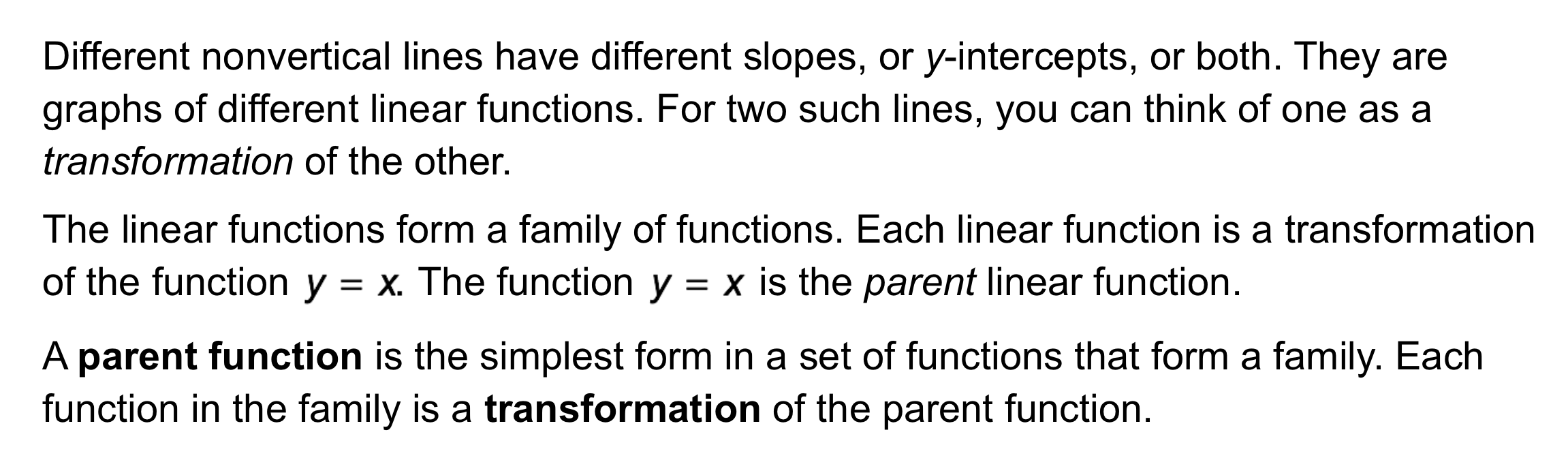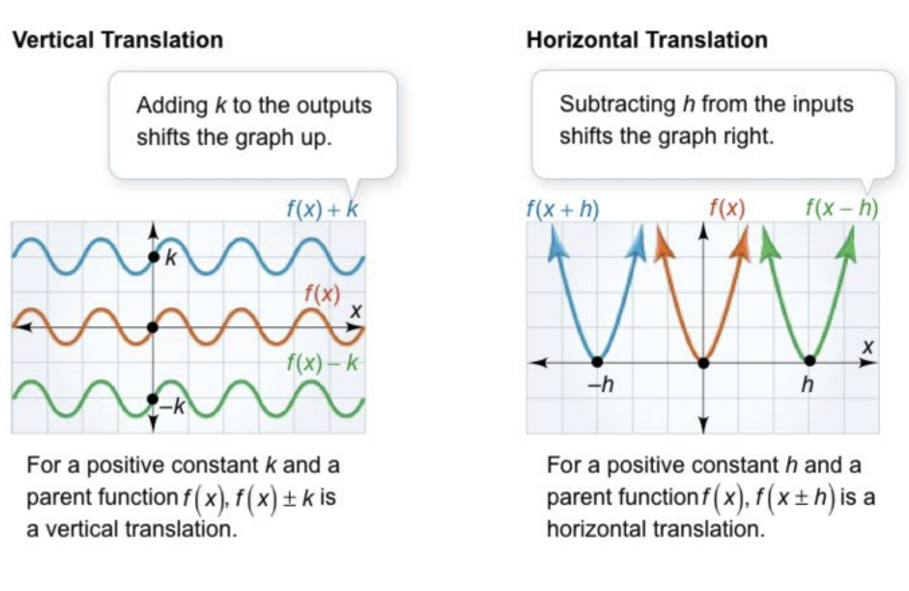3
4
5
3
10
Take Note: Define parent function.
4
10
Take Note: Define transformation of the graph of a function.
5
10
Take Note: Define vertical translation and horizontal translation.
6
10
Problem 1 Got It? How are the functions related?

Each output for y = 2x - 3 is three more than the corresponding output for y = 2x.
Each output for y = 2x - 3 is three less than the corresponding output for y = 2x.
7
10
Problem 1 Got It? How are the graphs of the functions related?

The graph of y = 2x - 3 is the graph of y = 2x translated left 3 units.
The graph of y = 2x - 3 is the graph of y = 2x translated up 3 units.
The graph of y = 2x - 3 is the graph of y = 2x translated right 3 units.
The graph of y = 2x - 3 is the graph of y = 2x translated down 3 units.
8
10
Problem 1 Got It? Graph the functions on the same coordinate plane. Zoom and pan your graph to establish an appropriate viewing window. After graphing, you may edit your responses to the previous 2 items.

9
10
Problem 2 Got It? Consider the projectile altitude f(x) of the airplane shown in Problem 2.

Suppose the flight leaves 30 minutes early. What function represents this transformation?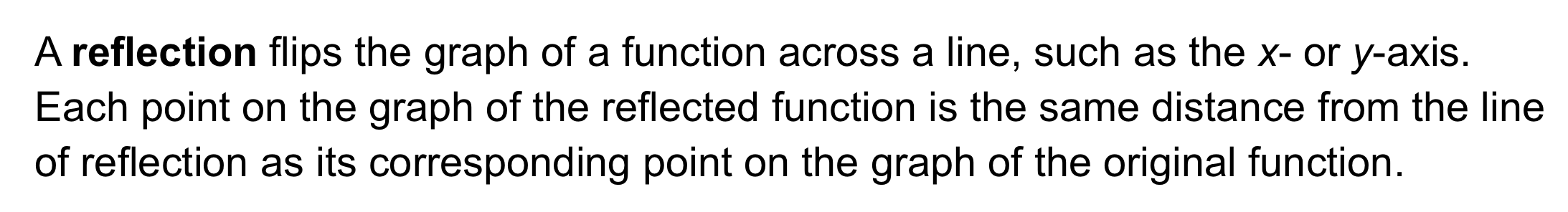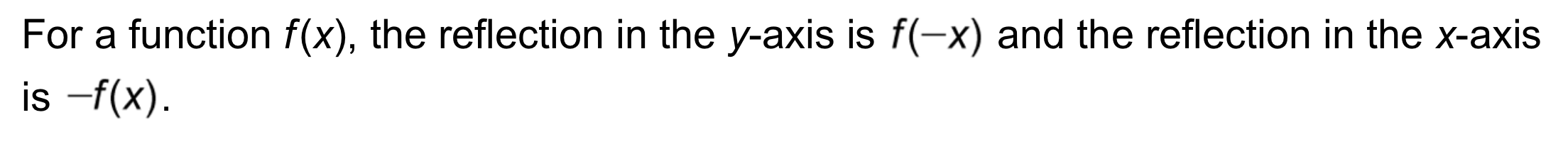10
11
12
13
14
10
10
Take Note: Define reflection as it pertains to graphs of functions.
11
5
Take Note: What does it mean to reflect a graph in the x-axis? You may use the canvas to illustrate your explanation.
12
5
Take Note: What does it mean to reflect a graph in the y-axis? You may use the canvas to illustrate your explanation.
13
5
Take Note: Which of the following functions represents a reflection in the y-axis of the function f(x)?
f(x-1)
f(-x)
-f(x)
f(x)-1
14
5
Take Note: Which of the following functions represents a reflection in the x-axis of the function f(x)?
f(x-1)
f(-x)
-f(x)
f(x)-115
15
10
Problem 3 Got It?
A
B
C
D16
17
18
16
10
Take Note: Define vertical stretch and vertical compression.
17
10
Take Note: Which of the following functions represents a vertical stretch of the function y=f(x)?
y=2f(x)
y=f(2x)
y=\frac{1}{2}f(x)
y=f(\frac{1}{2}x)
18
10
Take Note: Which of the following functions represents a vertical compression of the function y=f(x)?
y=2f(x)
y=f(2x)
y=\frac{1}{2}f(x)
y=f(\frac{1}{2}x)
19
10
Problem 4 Got It? For the function f(x) shown in Problem 4 and in the table below, what is the corresponding table for the transformation h(x)? Complete the table for h(x) on the canvas. Use a color other than black.

20
10
Problem 4 Got It? Reasoning: If several transformations are applied to a graph, will changing the order of transformations change the resulting graph? Explain.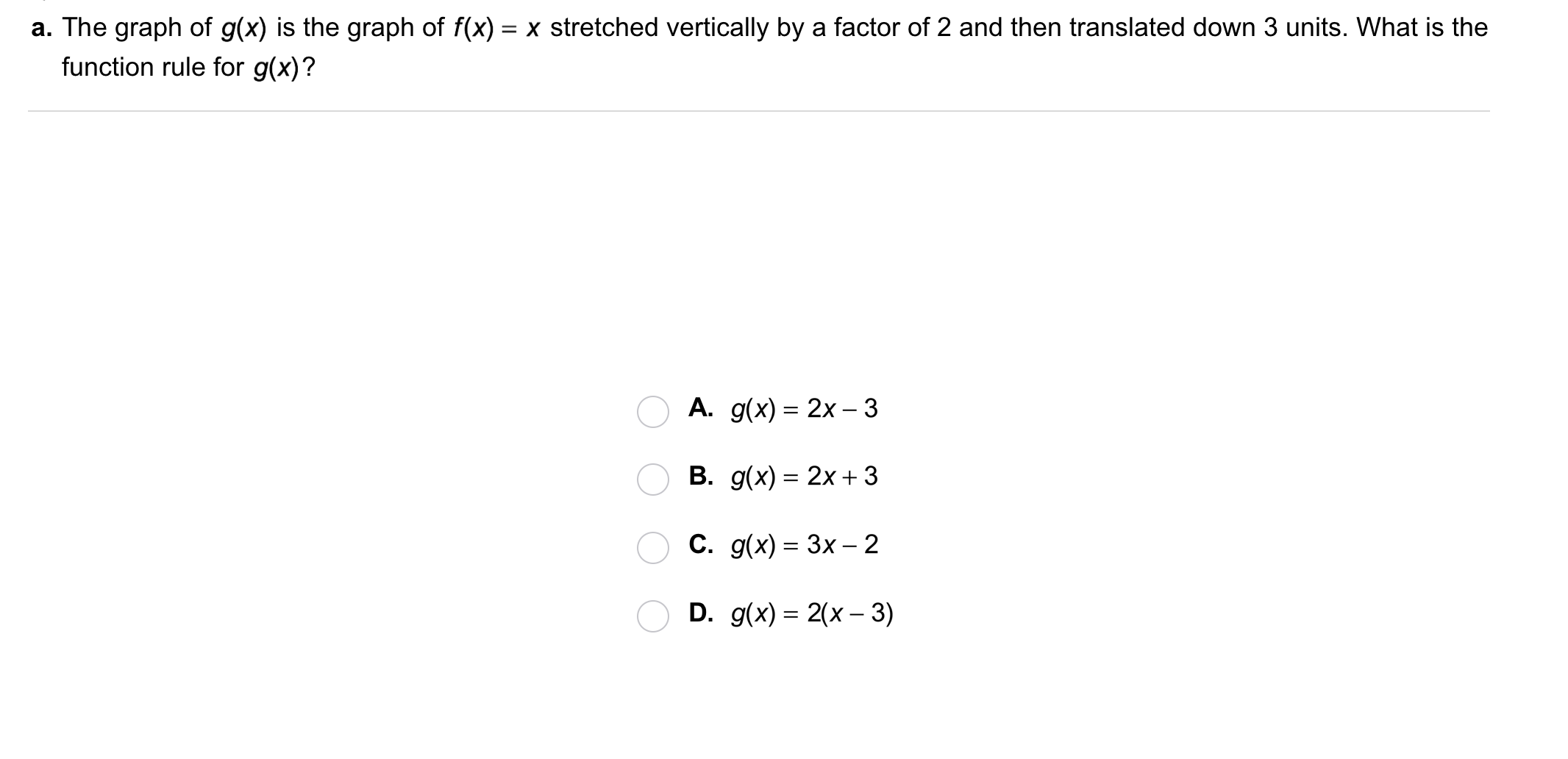21
21
10
Problem 5 Got It?
A
B
C
D22
22
10
Problem 5 Got It?
A
B
C
D23
23
10
Take Note: Summarize the mathematical content of this lesson. What topics, ideas, and vocabulary were introduced?